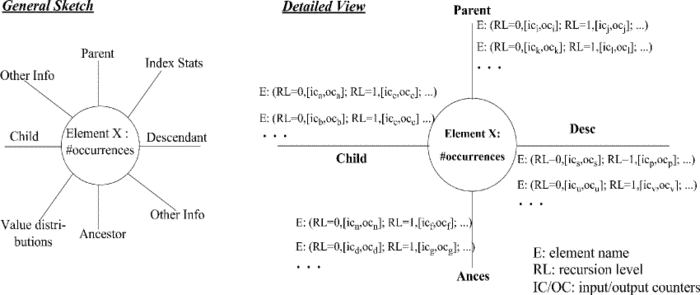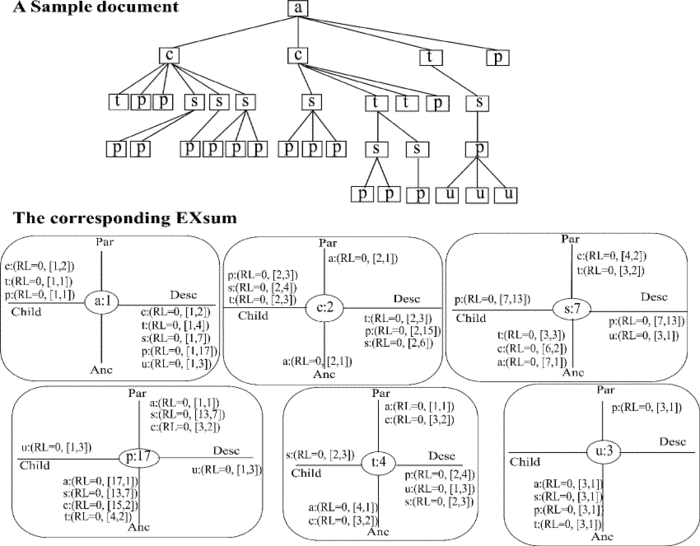# Statistics

Efficient database-driven XML processing relies on statistics to optimize and execute the (X)query in the best possible way. We developed the EXsum framework to have a succinct representation of meaningful XML document statistics that are made available to the cost-based query optimizer.

## Gathering Statistics on XML Documents

EXsum (Element-centered XML Summary) is a CAS (content-and-structure) summary, currently available in XTC, gathers the best summary characteristics (e.g., fast summary access, low memory footprint, and good accuracy and coverage), by inaugurating a new way of summarizing a document. Putting aside the strict document hierarchy, EXsum captures CAS distributions on an element/attribute name basis and, additionally, records distributions in relationships between an element name and its (possibly all) axes (e.g., child, parent, ancestor, and descendant). EXsum is, thus, a (disjoint) set of ASPE (axes summary per element) nodes and each ASPE node corresponds to a distinct element/attribute name in the XML document. An ASPE node, in turn, may have several spokes, each representing an axis relationship concerning to this element and a text spoke to capture content (value) distributions, as sketched in the following figure ("General Sketch").EXsum records, for each relationship, the fan-in (IC counter) and the fan-out (OC counter), enabling, therefore, the estimation of arbitrary query expressions (see figure "Detailed View"). Moreover, if the document presents structural recursion, EXsum captures such information through the RL (recursion level) counter. It is easy to see the feature of extensibility of EXsum. Whenever the user (or database administrator) needs more statistical information, a new spoke is added to the ASPE nodes.

## Example

The estimation process of EXsum is simple and based on an interpolation strategy. Given path expression //c/s/p, posed on the sample doument, EXsum loads only the ASPE nodes of c, s and p, corresponding to the location steps in the expression. Therefore, the memory footprint needed to estimate a path expression is bounded to the number of its steps, yielding, thus, a low memory footprint (i.e., the complete EXsum structure does not need to be loaded into memory).

The building principle of EXsum guarantees that two-step expressions are accurately estimated. In case of  n-step (n>2) expressions, an interpolation strategy is applied by decomposing the path expression into overlapping two-location-step fractions. For example, when estimating //c/s/p, we follow the child spoke of ASPE(c) to evaluate the first two location steps (//c/s) and obtain cardinality 4 (see OC counter in the figure). To evaluate the subsequent location step (/p), we use the overlapping fraction s/p, access ASPE(s), and look at the child spoke for a p, yielding a cardinality of 13. The interpolation factor is, thus, ASPE(s), which gives us a 7. Therefore, the estimated cardinality of the expression is 4/7*(13).For the estimation of value predicates in path expressions, EXsum allows, in a flexible way, the application of (possibly several) compression techniques (e.g., histograms, pruned suffix tress, etc) in the text spoke. Because of that, EXsum currently supports predicates with binary comparisons (e.g., =, >, <, etc.) for numeric values, the XPath function contains(), and a limited form of the ft:contains function for string values. To estimate expressions containing predicates, the interpolation strategy is also applied.